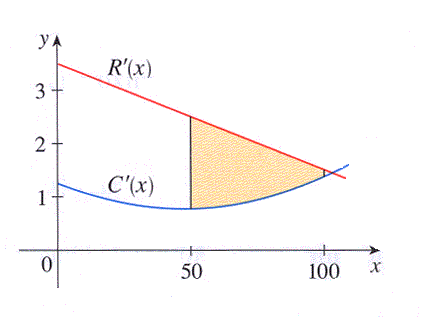Economists who study production of goods by a firm consider two functions. The revenue function $R(x)$ is the revenue the firm receives when x number of units are sold. The cost function $C(x)$ is the cost the firm incurs when producing x number of units. The derivatives of these functions $R'(x)$ and $C'(x)$ are called by economists the marginal revenue and cost function. The figure shows graphs of the marginal revenue function $R'$ and the marginal cost function $C'$ for a manufacturer. Assume that $R$ and $C$ are measured in thousands of dollars.(a) What does the shaded area in the figure represent?

(b) Upon closer inspection of the graph, a table of data values for $R'$ and $C'$ (each in thousands of dollars per unit manufactured) is generated below:

Use the Midpoint Rule with $n = 5$ to estimate the value of the shaded region (in thousands of dollars).

Value of the shaded region = thousand dollars

You can earn partial credit on this problem.